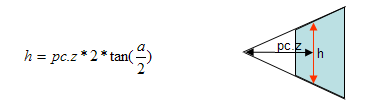Prev: Radar Approach - Testing Points Next: Implementation

Up to this point the required computations to compute the value of p in the camera referential coordinates, pc have been detailed. It is also assumed at this point that the pc.z has a value that is between nearDist and farDist, so its time to check the other coordinates, namely pc.y and pc.x.

Consider now a side view of the frustum in 2D to simplify the diagram. The horizontal arrow shows the value of pc.z. At that distance from the camera, pc.z the view frustum has a height h.where a is the vertical field of view angle.

This implies that, for the Y coordinate of pc to be inside the view frustum,

`	-h/2 < pc.y <  h/2`

or, in algorithmic style,

```	if (-h/2 > pc.y || pc.y  >  h/2)
return(OUTSIDE)```

The width of the frustum can be computed based on the height as

`	w = h * ratio;`

So the X component of pc can be tested with the following if statement:

```	if (-w/2 > pc.x || pc.x  >  w/2)
return(OUTSIDE)```

The following figure, although a little bit complex ( ;-> ) provides some information about the components of pc.This method should be faster than the previous approaches where six planes are tested. In here, only a dot product is performed for each pair of planes, whereas in the previous approaches a dot product was performed for every plane, in the worst case scenario. See the next section for an implementation.

 Prev: Radar Approach - Testing Points Next: Implementation

This site uses Akismet to reduce spam. Learn how your comment data is processed.